## Lena rolled two number cubes and recorded the results. What is the experimental probability that the sum of the next two numbers rolled is g

Question

Lena rolled two number cubes and recorded the results. What is the experimental probability that the sum of the next two numbers rolled is greater than 5? Please help

in progress 0
2 weeks 2021-09-01T13:39:19+00:00 1 Answers 0 views 0

1. Given:

Lena rolled two number cubes and recorded the results.

To find:

The experimental probability that the sum of the next two numbers rolled is greater than 5.

Solution:

If two number cubes rolled, then the total possible outcomes are:

(1,1), (1,2), (1,3), (1,4), (1,5), (1,6), (21,1), (2,2), (2,3), (2,4), (2,5), (2,6),

(3,1), (3,2), (3,3), (3,4), (3,5), (3,6), (4,1), (4,2), (4,3), (4,4), (4,5), (4,6),

(5,1), (5,2), (5,3), (5,4), (5,5), (5,6), (6,1), (6,2), (6,3), (6,4), (6,5), (6,6)

Total number of possible outcomes = 36

The possible cases in which the sum is less than or equal to 5 are (1,1), (1,2), (1,3), (1,4), (2,1), (2,2), (2,3), (3,1), (3,2), (4,1).

In 10 cases the sum is less than or equal to 5. So, the number of cases in which the sum is greater than 5 is: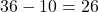So, the number of favorable outcomes = 26.

Now, the experimental probability that the sum of the next two numbers rolled is greater than 5 is: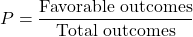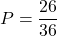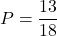Therefore, the required probability is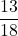.